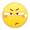## var chakhsu = function(r) { function t() { return b[Math.floor(Math.random() * b.length)] } function e() { return String.fromCharCode(94 * Math.random() + 33) } function n(r) { for (var n = document.createDocumentFragment(), i = 0; r > i; i++) { var l = document.createElement("span"); l.textContent = e(), l.style.color = t(), n.appendChild(l) } return n } function i() { var t = o[c.skillI]; c.step ? c.step--:(c.step = g, c.prefixP < l.length ? (c.prefixP >= 0 && (c.text += l[c.prefixP]), c.prefixP++) : "forward" === c.direction ? c.skillP < t.length ? (c.text += t[c.skillP], c.skillP++) : c.delay ? c.delay--:(c.direction = "backward", c.delay = a) : c.skillP > 0 ? (c.text = c.text.slice(0, -1), c.skillP--) : (c.skillI = (c.skillI + 1) % o.length, c.direction = "forward")), r.textContent = c.text, r.appendChild(n(c.prefixP < l.length ? Math.min(s, s + c.prefixP) : Math.min(s, t.length - c.skillP))), setTimeout(i, d) } /*以下内容自定义修改*/ var l = "SAMA主题开发记录", o = ["", ].map(function(r) { return r + "" }), a = 2, g = 1, s = 5, d = 75, b = ["rgb(110,64,170)", "rgb(150,61,179)", "rgb(191,60,175)", "rgb(228,65,157)", "rgb(254,75,131)", "rgb(255,94,99)", "rgb(255,120,71)", "rgb(251,150,51)", "rgb(226,183,47)", "rgb(198,214,60)", "rgb(175,240,91)", "rgb(127,246,88)", "rgb(82,246,103)", "rgb(48,239,130)", "rgb(29,223,163)", "rgb(26,199,194)", "rgb(35,171,216)", "rgb(54,140,225)", "rgb(76,110,219)", "rgb(96,84,200)"], c = { text: "", prefixP: -s, skillI: 0, skillP: 0, direction: "forward", delay: a, step: g }; i() }; chakhsu(document.getElementById('chakhsu'));

2018-03-17 4 个评论 551 字数

### 目前正在开发：

footer区域

#### BB几句：

Typecho随机彩色标签：下一篇

1.清秋暖冬 Windows XP @ Google Chrome 58.0.3029.110 April 23rd, 2018 at 01:03 pm

大佬主题很棒哟！1.碧池喵 Windows 7 @ Google Chrome 65.0.3325.181 April 24th, 2018 at 03:06 pm

假的都是假的2.无用挂件 Android @ Google Chrome 55.0.2883.91 April 5th, 2019 at 09:49 pm

用Bootstrap的果然都是大神...主题真心好看:-)

1.碧池喵 Windows @ Google Chrome 72.0.3626.96 April 9th, 2019 at 07:06 pm

噗 没完成鸭 早就咕咕咕了